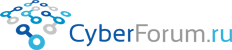IGPIGP
 Войти Регистрация Восстановить пароль
 Правила Блоги Социальные группы Поиск Сообщения за день Все разделы прочитаны
Оценить эту запись

# About relativistic mass - gravitational mass

Запись от IGPIGP размещена 16.05.2020 в 14:00
Обновил(-а) IGPIGP 29.05.2020 в 18:08

 Relativistic gravitational mass is the result of the Lorentz transformation for mass as a charge of the gravitational field. This equation can be got in almost one line. Let there be two bodies - balls with rest masses m0 located at the non-coincident points A and B. Each ball is rigidly attached to side of a spoke - a ruler. On the opposite end of the spoke against to a ball is an observer. The spokes are directed at each other's balls, so that the balls are outside (left and right) and the observers inside are between the balls. The two observers described are aware of the pattern of experiment and trust its organizers. They was told: 1 You will start moving towards each other according to the signal emithed from the equally distanced point 0 placed on a straight line connecting you, synchronously accelerating (the acceleration programs are embedded in the engines system and work the same in local time). 2 Having accelerated to v0, the engines will turn off and the movement to 0 and each other will continue by inertia. That is, - I repeat, - the body-ruler system resembles a spoke consisting of a massive ball (body m0) rigidly fixed at the end of a thin, weightless rod directed along a line of movement. The surface of each rod is calibrated in mm. The distance between the ends of the spokes is sufficient for acceleration and inertia until the moment when the moment of mutual contact of the spokes of each of the participants (and the meeting of the participants - observers) is fixed in the system of point 0 (system of the center of mass of the system of 2 bodies mo). This will happen at point 0. There is one more important condition. Somewhere far ( at a distance H >> | AB | ) on the axis of symmetry in the center mass system of the perpendicular line of of moving, there is a massive body. It creates a uniform gravitational field distributed along the entire line of moving from A to B. The vector of strength of the field is directed perpendicular to the velocities of both bodies at any moment of time. Denote the strength of this field G. We describe the observation of an observer from the system of the left ball after the finish of its acceleration to a speed v0 at the moment of time of meeting with his partner at the point 0. Let the length of each spoke in its reference frame be Lo. This is, at the moment of meeting with his partner, the left observer is sure that the distance from his ball to point 0 is equal to L0. The observer records the moment of rotation for the gravitational force acting on his ball relative to point 0:Further, he tries to record the same moment for his twin, flying towards. The distance from the aproaching ball to point 0 is the shoulder of force - L, the mass of the twin - M, the of gravity field strength (with good accuracy) - G. Total:The shoulder is measured in the observer system (left), it is reduced in accordance with the Lorentz transformation:And this means that for J0 = J it is necessary that:Where u is the speed of approach of the participants one to other and it is the relativistic sum of the speeds v0 and v0:That is, the expression:obtained from the condition of symmetry with respect to gravity using the masses as charges of the gravitational field to determine the interaction. This means that the obtained M (m, v) is, in fact, the Lorentz transformation for the gravitational mass with respect to speed. This expression does not differ from the expression obtained for the inertial mass: https://www.cyberforum.ru/blogs/212894/blog6161.html where symmetry with respect to momentum is used in a system of 2 approaching bodies. The coincidence of these results is quite natural and it proves the equivalence of gravitational and inertial relativistic masses. The assertion that there is no relativistic mass as a concept in SRT is not compatible with logic. Either they both are true, or there neither relativistic mass nor SRT as a theory is true.
Размещено в Theory of relativity
Просмотров 136 Комментарии 0
Всего комментариев 0
Комментарии
 КиберФорум - форум программистов, компьютерный форум, программирование Реклама - Обратная связь